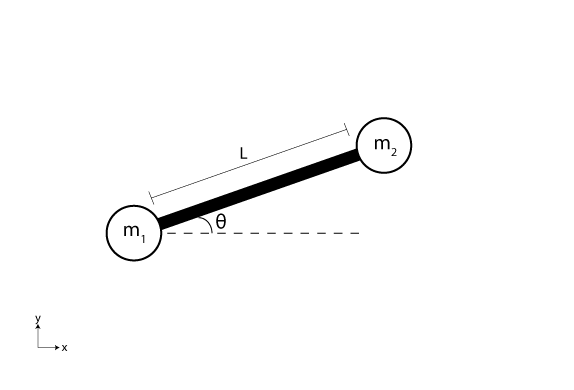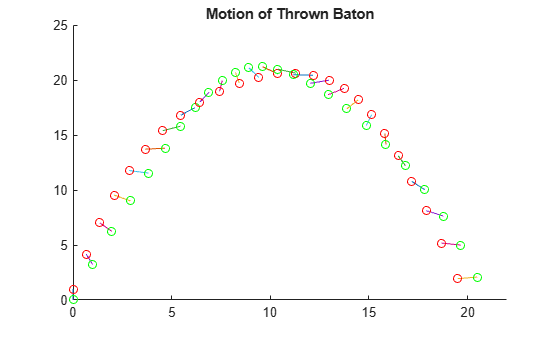# Solve Equations of Motion for Baton Thrown into Air

This example solves a system of ordinary differential equations that model the dynamics of a baton thrown into the air . The baton is modeled as two particles with masses ${\mathit{m}}_{1}$ and ${\mathit{m}}_{2}$ connected by a rod of length $\mathit{L}$. The baton is thrown into the air and subsequently moves in the vertical xy-plane subject to the force due to gravity. The rod forms an angle $\theta$ with the horizontal and the coordinates of the first mass are $\left(\mathit{x},\mathit{y}\right)$. With this formulation, the coordinates of the second mass are $\left(\mathit{x}+\mathit{L}\text{\hspace{0.17em}}\mathrm{cos}\text{\hspace{0.17em}}\theta ,\mathit{y}+\mathit{L}\text{\hspace{0.17em}}\mathrm{sin}\text{\hspace{0.17em}}\theta \right)$.The equations of motion for the system are obtained by applying Lagrange's equations for each of the three coordinates, $\mathit{x}$, $\mathit{y}$, and $\theta$:

`$\begin{array}{l}\left({\mathit{m}}_{1}+{\mathit{m}}_{2}\right)\stackrel{¨}{\mathit{x}}-{\mathit{m}}_{2}\mathit{L}\text{\hspace{0.17em}}\stackrel{¨}{\theta }\text{\hspace{0.17em}}\mathrm{sin}\text{\hspace{0.17em}}\theta -{\mathit{m}}_{2}\mathit{L}\text{\hspace{0.17em}}{\stackrel{˙}{\theta }}^{2}\text{\hspace{0.17em}}\mathrm{cos}\text{\hspace{0.17em}}\theta =0,\\ \left({\mathit{m}}_{1}+{\mathit{m}}_{2}\right)\stackrel{¨}{\mathit{y}}-{\mathit{m}}_{2}\mathit{L}\text{\hspace{0.17em}}\stackrel{¨}{\theta }\text{\hspace{0.17em}}\mathrm{cos}\text{\hspace{0.17em}}\theta -{\mathit{m}}_{2}\mathit{L}\text{\hspace{0.17em}}{\stackrel{˙}{\theta }}^{2}\text{\hspace{0.17em}}\mathrm{sin}\text{\hspace{0.17em}}\theta +\left({\mathit{m}}_{1}+{\mathit{m}}_{2}\right)\mathit{g}=0,\\ {\mathit{L}}^{2}\stackrel{¨}{\theta }-\mathit{L}\text{\hspace{0.17em}}\stackrel{¨}{\mathit{x}}\text{\hspace{0.17em}}\mathrm{sin}\text{\hspace{0.17em}}\theta +\mathit{L}\text{\hspace{0.17em}}\stackrel{¨}{\mathit{y}}\text{\hspace{0.17em}}\mathrm{cos}\text{\hspace{0.17em}}\theta +\mathit{g}\text{\hspace{0.17em}}\mathit{L}\text{\hspace{0.17em}}\mathrm{cos}\text{\hspace{0.17em}}\theta =0.\end{array}$`

To solve this system of ODEs in MATLAB®, code the equations into a function before calling the solver `ode45`. You can either include the required functions as local functions at the end of a file (as done here), or save them as separate, named files in a directory on the MATLAB path.

### Code Equations

The `ode45` solver requires the equations to be written in the form $\stackrel{˙}{\mathit{q}}=\mathit{f}\left(\mathit{t},\mathit{q}\right)$, where $\stackrel{˙}{\mathit{q}}$ is the first derivative of each coordinate. In this problem, the solution vector has six components: $\mathit{x}$, $\mathit{y}$, the angle $\theta$, and their first derivatives:

`$\mathit{q}=\left[\begin{array}{c}{\mathit{q}}_{1}\\ {\mathit{q}}_{2}\\ {\mathit{q}}_{3}\\ {\mathit{q}}_{4}\\ {\mathit{q}}_{5}\\ {\mathit{q}}_{6}\end{array}\right]=\left[\begin{array}{c}\mathit{x}\\ \stackrel{˙}{\mathit{x}}\\ \mathit{y}\\ \stackrel{˙}{\mathit{y}}\\ \theta \\ \stackrel{˙}{\theta }\end{array}\right].$`

With this notation, you can rewrite the equations of motion entirely in terms of the elements of $\mathit{q}$:

`$\begin{array}{l}\left({\mathit{m}}_{1}+{\mathit{m}}_{2}\right)\stackrel{˙}{{\mathit{q}}_{2}}-{\mathit{m}}_{2}\mathit{L}\text{\hspace{0.17em}}\stackrel{˙}{{\mathit{q}}_{6}}\text{\hspace{0.17em}}\mathrm{sin}\text{\hspace{0.17em}}{\mathit{q}}_{5}={\mathit{m}}_{2}\mathit{L}\text{\hspace{0.17em}}{\mathit{q}}_{6}^{2}\mathrm{cos}\text{\hspace{0.17em}}{\mathit{q}}_{5},\\ \left({\mathit{m}}_{1}+{\mathit{m}}_{2}\right)\stackrel{˙}{{\mathit{q}}_{4}}-{\mathit{m}}_{2}\mathit{L}\text{\hspace{0.17em}}\stackrel{˙}{{\mathit{q}}_{6}}\text{\hspace{0.17em}}\mathrm{cos}\text{\hspace{0.17em}}{\mathit{q}}_{5}={\mathit{m}}_{2}\mathit{L}\text{\hspace{0.17em}}{\mathit{q}}_{6}^{2}\text{\hspace{0.17em}}\mathrm{sin}\text{\hspace{0.17em}}{\mathit{q}}_{5}-\left({\mathit{m}}_{1}+{\mathit{m}}_{2}\right)\mathit{g},\\ {\mathit{L}}^{2}\stackrel{˙}{{\mathit{q}}_{6}}-\mathit{L}\text{\hspace{0.17em}}\stackrel{˙}{{\mathit{q}}_{2}}\text{\hspace{0.17em}}\mathrm{sin}\text{\hspace{0.17em}}{\mathit{q}}_{5}+\mathit{L}\text{\hspace{0.17em}}\stackrel{˙}{{\mathit{q}}_{4}}\text{\hspace{0.17em}}\mathrm{cos}\text{\hspace{0.17em}}{\mathit{q}}_{5}=-\mathit{g}\text{\hspace{0.17em}}\mathit{L}\text{\hspace{0.17em}}\mathrm{cos}\text{\hspace{0.17em}}{\mathit{q}}_{5}.\end{array}$`

Unfortunately, the equations of motion do not fit into the form $\stackrel{˙}{\mathit{q}}=\mathit{f}\left(\mathit{t},\mathit{q}\right)$ required by the solver, since there are several terms on the left with first derivatives. When this occurs, you must use a mass matrix to represent the left side of the equation.

With matrix notation, you can rewrite the equations of motion as a system of six equations using a mass matrix in the form $\mathit{M}\left(\mathit{t},\mathit{q}\right)\text{\hspace{0.17em}}\stackrel{˙}{\mathit{q}}=\mathit{f}\left(\mathit{t},\mathit{q}\right)$. The mass matrix expresses the linear combinations of first derivatives on the left side of the equation with a matrix-vector product. In this form, the system of equations becomes:

`$\left[\begin{array}{cccccc}1& 0& 0& 0& 0& 0\\ 0& {\mathit{m}}_{1}+{\mathit{m}}_{2}& 0& 0& 0& -{\mathit{m}}_{2}\mathit{L}\text{\hspace{0.17em}}\mathrm{sin}\text{\hspace{0.17em}}{\mathit{q}}_{5}\\ 0& 0& 1& 0& 0& 0\\ 0& 0& 0& {\mathit{m}}_{1}+{\mathit{m}}_{2}& 0& {\mathit{m}}_{2}\mathit{L}\text{\hspace{0.17em}}\mathrm{cos}\text{\hspace{0.17em}}{\mathit{q}}_{5}\\ 0& 0& 0& 0& 1& 0\\ 0& -\mathit{L}\text{\hspace{0.17em}}\mathrm{sin}\text{\hspace{0.17em}}{\mathit{q}}_{5}& 0& \mathit{L}\text{\hspace{0.17em}}\mathrm{cos}\text{\hspace{0.17em}}{\mathit{q}}_{5}& 0& {\mathit{L}}^{2}\end{array}\right]\left[\begin{array}{c}\stackrel{˙}{{\mathit{q}}_{1}}\\ \stackrel{˙}{{\mathit{q}}_{2}}\\ \stackrel{˙}{{\mathit{q}}_{3}}\\ \stackrel{˙}{{\mathit{q}}_{4}}\\ \stackrel{˙}{{\mathit{q}}_{5}}\\ \stackrel{˙}{{\mathit{q}}_{6}}\end{array}\right]=\left[\begin{array}{c}{\mathit{q}}_{2}\\ {\mathit{m}}_{2}\mathit{L}\text{\hspace{0.17em}}{\mathit{q}}_{6}^{2}\mathrm{cos}\text{\hspace{0.17em}}{\mathit{q}}_{5}\\ {\mathit{q}}_{4}\\ {\mathit{m}}_{2}\mathit{L}\text{\hspace{0.17em}}{\mathit{q}}_{6}^{2}\text{\hspace{0.17em}}\mathrm{sin}\text{\hspace{0.17em}}{\mathit{q}}_{5}-\left({\mathit{m}}_{1}+{\mathit{m}}_{2}\right)\mathit{g}\\ {\mathit{q}}_{6}\\ -\mathit{g}\text{\hspace{0.17em}}\mathit{L}\text{\hspace{0.17em}}\mathrm{cos}\text{\hspace{0.17em}}{\mathit{q}}_{5}\end{array}\right]$`

From this expression, you can write a function that calculates the nonzero elements of the mass matrix. The function takes three inputs: $\mathit{t}$ and the solution vector $\mathit{q}$ are required (you must specify these inputs even if they are not used in the body of the function), and $\mathit{P}$ is an optional extra input used to pass in parameter values. To pass the parameters for this problem to the function, create `P` as a structure that holds the parameter values and then use the technique described in Parameterizing Functions to pass the structure to the function as an extra input.

```function M = mass(t,q,P) % Extract parameters m1 = P.m1; m2 = P.m2; L = P.L; g = P.g; % Mass matrix function M = zeros(6,6); M(1,1) = 1; M(2,2) = m1 + m2; M(2,6) = -m2*L*sin(q(5)); M(3,3) = 1; M(4,4) = m1 + m2; M(4,6) = m2*L*cos(q(5)); M(5,5) = 1; M(6,2) = -L*sin(q(5)); M(6,4) = L*cos(q(5)); M(6,6) = L^2; end ```

Next, you can write a function for the right side of each of the equations in the system $\mathit{M}\left(\mathit{t},\mathit{q}\right)\text{\hspace{0.17em}}\stackrel{˙}{\mathit{q}}=\mathit{f}\left(\mathit{t},\mathit{q}\right)$. Like the mass matrix function, this function takes two required inputs for $\mathit{t}$ and $\mathit{q}$, and one optional input for parameter values $\mathit{P}$.

```function dydt = f(t,q,P) % Extract parameters m1 = P.m1; m2 = P.m2; L = P.L; g = P.g; % Equation to solve dydt = [q(2) m2*L*q(6)^2*cos(q(5)) q(4) m2*L*q(6)^2*sin(q(5))-(m1+m2)*g q(6) -g*L*cos(q(5))]; end ```

### Solve System of Equations

First, create a structure `P` of parameter values for ${\mathit{m}}_{1}$, ${\mathit{m}}_{2}$, $\mathit{g}$, and $\mathit{L}$ by setting appropriately named fields in a structure. The structure `P` is passed to the mass matrix and ODE functions as an extra input.

```P.m1 = 0.1; P.m2 = 0.1; P.L = 1; P.g = 9.81```
```P = struct with fields: m1: 0.1000 m2: 0.1000 L: 1 g: 9.8100 ```

Create a vector with 25 points between 0 and 4 for the time span of the integration. When you specify a vector of times, `ode45` returns interpolated solutions at the requested times.

`tspan = linspace(0,4,25);`

Set the initial conditions of the problem. Since the baton is thrown upward at an angle, use nonzero values for the initial velocities, $\stackrel{˙}{{\mathit{x}}_{0}}=4$, $\stackrel{˙}{{\mathit{y}}_{0}}=20$, and $\stackrel{˙}{{\theta }_{0}}=2$. For the initial positions, the baton begins in an upright position, so ${\theta }_{0}=-\pi /2$, ${\mathit{x}}_{0}=0$, and ${\mathit{y}}_{0}=\mathit{L}$.

`y0 = [0; 4; P.L; 20; -pi/2; 2];`

Use `odeset` to create an options structure that references the mass matrix function. Since the solver expects the mass matrix function to have only two inputs, use an anonymous function to pass in the parameters `P` from the workspace.

`opts = odeset('Mass',@(t,q) mass(t,q,P));`

Finally, solve the system of equations using `ode45` with these inputs:

• An anonymous function for the equations `@(t,q) f(t,q,P)`

• The vector `tspan` of times where the solution is requested

• The vector `y0` of initial conditions

• The options structure `opts` that references the mass matrix

`[t,q] = ode45(@(t,q) f(t,q,P),tspan,y0,opts);`

### Plot Results

The outputs from `ode45` contain the solutions of the equations of motion at each requested time step. To examine the results, plot the motion of the baton over time.

Loop through each row of the solution, and at each time step, plot the position of the baton. Color each end of the baton differently so that you can see its rotation over time.

```figure title('Motion of a Thrown Baton, Solved by ODE45'); axis([0 22 0 25]) hold on for j = 1:length(t) theta = q(j,5); X = q(j,1); Y = q(j,3); xvals = [X X+P.L*cos(theta)]; yvals = [Y Y+P.L*sin(theta)]; plot(xvals,yvals,xvals(1),yvals(1),'ro',xvals(2),yvals(2),'go') end hold off```### References

 Wells, Dare A. Schaum’s Outline of Theory and Problems of Lagrangian Dynamics: With a Treatment of Euler’s Equations of Motion, Hamilton’s Equations and Hamilton’s Principle. Schaum's Outline Series. New York: Schaum Pub. Co, 1967.

### Local Functions

Listed here are the local helper functions that the ODE solver calls to calculate the solution. Alternatively, you can save these functions as their own files in a directory on the MATLAB path.

```function dydt = f(t,q,P) % Equations to solve % Extract parameters m1 = P.m1; m2 = P.m2; L = P.L; g = P.g; % RHS of system of equations dydt = [q(2) m2*L*q(6)^2*cos(q(5)) q(4) m2*L*q(6)^2*sin(q(5))-(m1+m2)*g q(6) -g*L*cos(q(5))]; end %------------------------------------------------ function M = mass(t,q,P) % Mass matrix function % Extract parameters m1 = P.m1; m2 = P.m2; L = P.L; g = P.g; % Set nonzero elements in mass matrix M = zeros(6,6); M(1,1) = 1; M(2,2) = m1 + m2; M(2,6) = -m2*L*sin(q(5)); M(3,3) = 1; M(4,4) = m1 + m2; M(4,6) = m2*L*cos(q(5)); M(5,5) = 1; M(6,2) = -L*sin(q(5)); M(6,4) = L*cos(q(5)); M(6,6) = L^2; end```